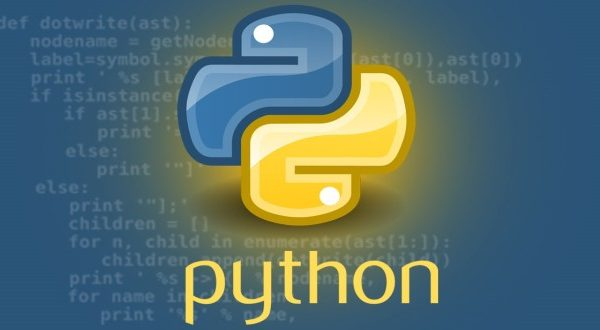# Gradient Boosting Classifiers in Python with Scikit-Learn### Introduction

Gradient boosting classifiers are a group of machine learning algorithms that combine many weak learning models together to create a strong predictive model. Decision trees are usually used when doing gradient boosting. Gradient boosting models are becoming popular because of their effectiveness at classifying complex datasets, and have recently been used to win many Kaggle data science competitions.

The Python machine learning library, Scikit-Learn, supports different implementations of gradient boosting classifiers, including XGBoost.

In this article we’ll go over the theory behind gradient boosting models/classifiers, and look at two different ways of carrying out classification with gradient boosting classifiers in Scikit-Learn.

### Defining Terms

Let’s start by defining some terms in relation to machine learning and gradient boosting classifiers.

To begin with, what is classification? In machine learning, there are two types of supervised learning problems: classification and regression.

Classification refers to the task of giving a machine learning algorithm features, and having the algorithm put the instances/data points into one of many discrete classes. Classes are categorical in nature, it isn’t possible for an instance to be classified as partially one